#lyceum Lectures
desu-cartes
June 20, 2018
Contents
1 Introduction to Manifolds 1
1.1 Topology . . . . . . . . . . . . . . . . . . . . . . . . . . . . . . . 2
1.2 Manifolds and smoothness . . . . . . . . . . . . . . . . . . . . . . 5
A A bit about continuity 10
1 Introduction to Manifolds
Date: December 1, 2017
Lecturer: Killingform
The goal for this short lecture will be to deﬁne a smooth manifold (whatever
that is!!!!). We rapidly begin building up elementary topology and then throw
the deﬁnition of manifold at the reader, and then we will elabourate on what
it means to be smooth. This will serve as the basis for further lectures on
diﬀerential forms and Lie groups.
Preamble
Euclidean space is the variety of coordinate space we deal most often with in our
day to day life. We envision the space we live in as a three-dimensional Euclidean
space where a point in space is represented as a triple, (x, y, z) R
3
. The key
idea about Euclidean space is that it is ‘ﬂat, where there is no general curvature
of the space as opposed to objects like the sphere or a donut. Euclidean space
is generalized to any dimension, being represented by the set of n-tuples of real
numbers, denoted R
n
.
The diﬃcult thing about manifolds is that they are like pornography: diﬃcult
to deﬁne, but you know it when you see it. Our working deﬁnition will be
that a manifold generalizes our notion of “Euclidean space” in that, though
1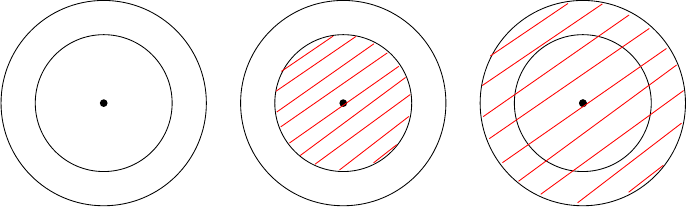looking globally, we might not immediately recognize it as ﬂat, if we zoom in
on the manifold, it will resemble closely to Euclidean space up to “continuous
deformation” such as stretching and moving (but no ripping!).
In order to discuss such continuous deformation, we need to develop a general
enough concept of continuity. We do this via topology. Topology deals with
all things stretching, shrinking, and growing. As long as it is a continuous
deformation, topology has something to say. What we ﬁrst will do is discuss
the notion of “closeness” which will help us better approximate what it means
to continuously deform a space into another.
1.1 Topology
Suppose we have a set X of points. We wish to discuss the notion of closeness
between these points. If we have a metric—that is, a distance function—we can
easily say what it means for points to be close together by discussing balls around
points, specifying certain radii of the balls. This is not as general as we require
for topology, as we would like to abandon the idea of a metric altogether. What
we ﬁrst do is we take our set X and specify a collection of “neighbourhoods”
(which will be subsets of X) in our set which characterize when points are
suﬃciently close to one-another. In a metric space, these neighbourhoods often
correspond with the aforementioned balls. Along with our English notion of
“neighbourhood” we have some expectations:
(i) If we have two neighbourhoods, their union forms another neighbourhood.
(ii) if we have two neighbourhoods, their intersection forms another neigh-
bourhood.
N
1
N
2
N
1
N
2
N
1
N
2
x
x
x
If N
1
and N
2
are both neighbourhoods of a point x, then
their intersection N
1
N
2
and union N
1
N
2
are also
both neighbourhoods of x.
These are reasonable expectations. We now formally deﬁne what it means by
a topology on the set X, hoping to draw a parallel between our expectations of
“neighbourhoods”, and what we deﬁne to be neighbourhoods.
2
Deﬁnition 1 (Topology on X). A topology on a set X is a collection of subsets
J such that:
(i) X, J;
(ii) Any arbitrary union of sets in J is again in J;
(iii) Any ﬁnite intersection of sets in J is again in J.
Together, we call (X, J) a topological space. Elements in J will be called open
sets of X. A few peculiarities arise in this deﬁnition. Demanding that the whole
set X is an open set is not too much to ask as it still agrees with preconceived
notions of neighbourhoods, but we also demand the empty set to be open. The
reason behind this is more of a corollary of formality. For now we can accept
it as it is, but the reason the empty set is open is because when we ﬁnd the
induced “topology” from a metric on a set, this is the case. Another peculiarity
is that in (ii) we suggest we can take any arbitrary union (that is, we can take
inﬁnite unions, or ﬁnite ones) of open sets and it will be again open, but only
ﬁnite intersections of open sets are open. This peculiarity also ﬁnds itself as a
result of the induced topology from a metric, but we will say a bit more on it
later.
Example 1. Consider the real number line, R. The topology on R is formed
by taking the collection of open intervals of the form (a, b) and extending this
set by adding all the arbitrary unions of sets of this form. That is, a set is open
in R if and only if it is a union of open intervals.
Note that R is the union of all the open intervals, and the empty set is the union
of no open intervals. It’s not diﬃcult to check the rest of the axioms of a topology
are satisﬁed. For a second, assume we are allowed to call inﬁnite intersections
of sets open. Consider the family of open sets F := {(1/n, 1/n) R | n > 1}.
Take the inﬁnite intersection across this family of open sets. You get that
T
F = {0}. This doesn’t seem to respect what we called neighbourhoods in R,
nor does it seem to respect our preconceptions of neighbourhoods. This gives
a glimpse into why we do not require inﬁnite intersections of open sets to be
open.
A deﬁnition I am not sure will come in handy:
Deﬁnition 2. A closed set in a topological space (X, J) is a subset K X
such that its complement K
C
:= {x X | x 6∈ K} is open.
Exercise 1. What are the closed sets in R according to our deﬁnition of its
standard topology above?
Now we will look at the idea of “continuous” functions between topological
spaces.
3
We won’t take for granted that you know what the epsilon-delta deﬁnition of
continuity is. But we can sketch the idea of continuity of a function f : X Y
between two topological spaces (we keep the topologies of these spaces implicit,
referring to “open in X and “open in Y ”) in terms of neighbourhoods as follows:
“The function f is continuous at x X if any neighbourhood of x gets mapped
to a neighbourhood of f(x).”
That is, if you stay close enough to x, you get mapped by f closely to f(x).
With some mathemagical trickery we make the following deﬁnition:
Deﬁnition 3 (Continuous function). Let X and Y be topological spaces. A
function f : X Y is continuous if the pre-image,
f
1
[O] := {x X | f (x) O},
of all open sets O in Y is open in X.
When considering R
n
, this coincides with your usual deﬁnition of continuity you
learn in analysis or calculus or whatever.
For those wondering about the mathemagical trickery, it is outlined in appendix
A (assuming you get the idea of a ”metric space”, but nothing more).
Exercise 2. Show that the identity function id: X X on any topological
space X deﬁned by id(x) = x, for all x X, is continuous.
Now the continuous deformation we wished to speak about in the beginning is
not quite just “continuous” functions. Consider the function g : [0, 2π) S
1
deﬁned by:
g(θ) = (cos θ, sin θ),
where S
1
denotes the unit circle. The function is continuous, and also has the
property of being bijective (meaning that it admits an inverse function, g
1
.
The function however seems to fail at preserving shape, as it eﬀectively deforms
the interval [0, 2π) (a line) into S
1
(a circle). That is, it glues one end of the
interval to the other! This is not an example of “continuous deformation” since
gluing doesn’t result from the bending, shrinking, and growing movements that
we have emphasized. So “continuous” is not enough!
The problem with g is that the inverse g
1
is discontinuous at the point (1, 0),
because every neighbourhood about (1, 0) on S
1
is the union of two intervals:
[0, a) (b, 2π),
which happens to be not be open when pulled back to S
1
, given what is called
the subspace topology inherited from R
2
(the subspace topology calls sets open
4
if there the set is a restriction of an open set in the larger topology, in this case
R
2
).
To ﬁx this sort of problem, we characterize continuous deformation using the
following type of function:
Deﬁnition 4 (Homeomorphism). A function f : X Y between topological
spaces is called a homeomorphism if it is a continuous bijection where its inverse
f
1
is also continuous.
Eﬀectively this allows us to continuous deform X into Y via f, and then undo
it using f
1
in a continuous way (which was impossible with the example of
g above). In the case that f : X Y is a homeomorphism, we say that X is
homeomorphic to Y .
Deﬁnition 5 (Locally Euclidean). A topological space X is said to be locally
Euclidean if for every x X there is an open set U in X and a function
ϕ: U R
n
such that:
(i) x U;
(ii) ϕ is a homeomorphism onto its image.
The pair (U, ϕ) is called a chart on X, the collection of charts constituting an
atlas on X.
Perhaps in more accessible language: a locally Euclidean space is one which has
a neighbourhood around each point that resembles Euclidean space in that we
can continuously deform the neighbourhood to look like it is ﬂat!
1.2 Manifolds and smoothness
We begin with a deﬁnition:
Deﬁnition 6 (Hausdorﬀ space). A topological space X is Hausdorﬀ if for any
two distinct points x, y X, there exists two disjoint, open sets U, V X such
that x U and y V .
Eﬀectively, the so-called Hausdorﬀ property provides a sort of degree of sep-
aration for a topological space, giving any two distinct points disjoint open
neighbourhoods. This is akin to Euclidean space, and it will play a subtle role
in the study of manifolds.
A little thing to note is that we are going to deﬁne what it means to be a “man-
ifold”, but there are several views on what a manifold should be. Some authors
choose to simply call a locally Euclidean space a topological manifold and that is
it. However, the majority of authors like to include a few more properties (such
5
as the Hausdorﬀ property) which give the structure of a manifold nicer-behaved
properties.
With this in mind, we apologize as now we are going to throw a large amount
of relatively-unmotivated terminology into the mix, in hopes that we can just
take them for granted and move forward to our goal.
Deﬁnition 7 (Basis for a topology). Let X be a set. A basis for a topology on
X is a collection B of subsets of X such that:
(i) For all x X there exists B B such that x B.
(ii) If x B
1
B
2
for B
1
, B
2
B, then there exists a B
3
B such that x B
3
and B
3
B
1
B
2
.
We deﬁne a topology J on X generated by B by: O J each x O has
a B B such that B O. So a basis for a topology gives us a simpler way
of describing a topology. For example, we almost used a basis to describe the
topology for R. A basis for this topology is the set of all open intervals.
Deﬁnition 8 (Second-countable). A topological space X is second-countable if
it admits a countable basis for its topology.
Deﬁnition 9 (Topological manifold). A topological manifold is a topological
space M which is:
(i) Locally Euclidean (that is, it has an atlas);
(ii) Hausdorﬀ;
(iii) Second-countable.
In the case each chart in the atlas of M maps into R
n
for a ﬁxed n, we say that
M has dimension n, or that it is an n-manifold, for short.
Now a few examples to take for granted. Feel free to try your hand at proving
that each is locally Euclidean:
1. Unsurprisingly, R
n
is an n-manifold.
2. The sphere, denoted by S
2
, is a 2-manifold.
3. The torus (or donut), denoted by T
2
, is a 2-manifold.
4. The circle, S
1
, is a 1-manifold.
5. The graph of a continuous function f : U R
n
where U R
m
is an m-
manifold. It has only one chart, being the projection on the ﬁrst coordinate
of the graph.
6. If M is an m-manifold and N is an n-manifold, then M ×N is an (m + n)-
manifold.
6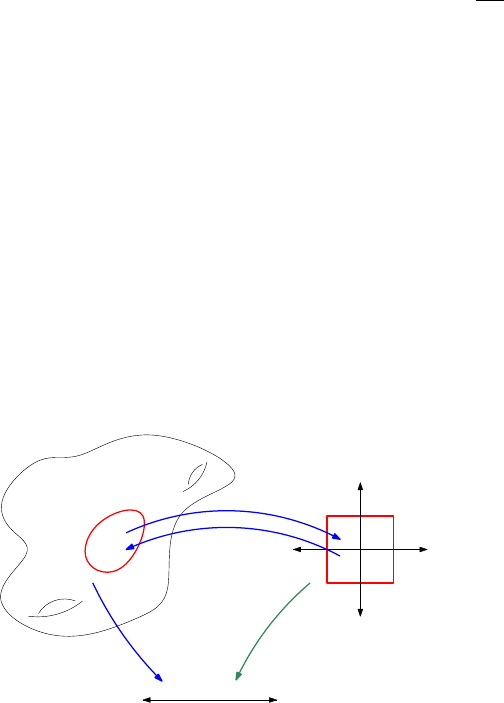Now that we know what manifolds are, we are without a goal. So let’s explore
what we can do with a manifold. Since it is locally Euclidean, what can we do
with Euclidean space that we might want to do in a more general scenario on a
manifold? The ﬁrst and only thing on the mind of any highschool or ﬁrst-year
student should be “calculus”. What would it take to do calculus on manifolds?
This is an excellent goal. So now we want to develop topological manifolds in
order to do calculus on them. If O is an open set in R
n
, a function g : O R
n
is called smooth if and only if g is inﬁnitely diﬀerentiable. That is,
n
g
x
n
exists
for all n.
For most people, it is more comfortable discussing maps O R than into R
n
,
so we will stick with that for now. If we have a map f : M R on a manifold
M, what might it mean for it to be “smooth”?
Since the domain of f is M , we cannot naively diﬀerentiate our function since
M is not necessarily R
n
—that is, it’s not always ﬂat. But what we do have is
the next best thing!
We have for any point p in M a neighbourhood U around p which we can
ﬂatten into an open subset of R
n
via a chart ϕ : U R
n
. So now we have two
ingredients, f and ϕ, and we need to combine them to hopefully make something
we can diﬀerentiate, and hence discuss the smoothness of. There is only one
sensible way of doing this, and that is by composing them:
f ϕ
1
: ϕ(U) R
where we take advantage of the fact that ϕ has an inverse ϕ
1
: ϕ(U) U .
f ϕ
1
ϕ
1
ϕ
f
ϕ(U)
M
R
n
R
Note that this is sort of our situation, we have made a function between R
n
and
R based on f and ϕ and now we can diﬀerentiate this composition (the green
function).
It now might be tempting to just deﬁne f as “smooth” at p if there exists such
a ϕ such that this composition f ϕ
1
is smooth in the usual sense. There
remains a problem however: it might depend on the chart, ϕ!
7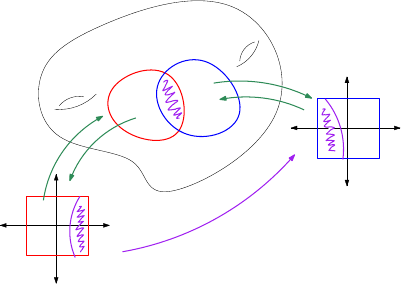Suppose that ψ : V R
n
is another chart such that p V . We could equally
call f (not) smooth if f ψ
1
is (resp. not) smooth. Note that with a little
algebra of functions we get:
f ψ
1
= f (ϕ
1
ϕ) ψ
1
= (f ϕ
1
) (ϕ ψ
1
),
on ϕ(U V ), which is not always smooth in the usual sense of the word.
We would perhaps need both parenthetical parts f ϕ
1
and ϕ ψ
1
to be
smooth. It’s not hard to imagine a case where you can choose the charts so that
only one of these compositions is smooth. In this way, our topological manifolds
are not quite suited for discussing smootheness—not yet at least!
To make this working deﬁnition of smoothness not depend on charts, we can
force the smoothness of ϕ ψ
1
using the following deﬁnition:
Deﬁnition 10 (Compatible charts). Let M be a topological manifold. Two
charts (U, ϕ) and (V, ψ) are said to be compatible if
ψ ϕ
1
: ϕ(U V ) ψ(U V )
is a diﬀeomorphism (that is, it is bijective and both it and its inverse are
smooth).
In this manner, if we have an atlas consisting of compatible charts, the smooth-
ness of a function f : M R does not depend on the chart we choose. If it is
smooth with respect to one chart, it is smooth with respect to all charts.
U
V
ψ
1
ψ
ψ ϕ
1
ϕ
1
ϕ
The purple function here is the one we require to be smooth in order for the
charts to be compatible.
Deﬁnition 11 (Smooth atlas). Let M be a topological manifold. An atlas A
on M is called a smooth atlas if A is an atlas (the usual kind) such that if ϕ
and ψ are charts in A, then they are compatible.
8
Now when we can conclusively deﬁne that a function f : M R is smooth at
p M if there exists a chart ϕ containing p such that f ϕ
1
is smooth in the
usual sense. That is, assuming M has a smooth atlas. Now we are not done
quite yet. Consider a manifold M with two smooth atlases, A and B := A {ψ}
for some chart ψ which is not in A. Are these atlases inherently diﬀerent from
each other? If f : M R is a function on M, we can consider its smoothness
at some p M which has ϕ A and ψ B as charts around p:
f ϕ
1
is smooth f ψ
1
= (f ϕ
1
) (ϕ ψ
1
) is smooth
, and hence adding a compatible chart ψ to A did not change which functions
end up being smooth.
In the spirit of this, we say that two smooth atlases are compatible if their
union is also a smooth atlas. The compatibility of smooth atlases gives us an
equivalence relation where we can discuss the smooth structure of the manifold
despite there being many smooth atlases that give the identical collection of
diﬀerentiable functions.
Theorem 1. Let M be a manifold with a smooth atlas A. Then there exists a
unique maximal smooth atlas M containing A (with respect to inclusion).
This maximally smooth atlas gives us a canonical choice as to what smooth
atlas to consider if any at all. And so now we have the following deﬁnition:
Deﬁnition 12 (Smooth manifold). A smooth manifold is a topological manifold
with a choice of maximal smooth atlas, called the smooth structure.
So now let’s forget about “topological manifolds” Whenever we talk about “man-
ifolds”, the “smooth” part will be assumed and implicit.
N.B.: one can consider other diﬀerentiable structures for manifolds where it is
only k-times diﬀerentiable rather than inﬁnitely diﬀerentiable.
Example 2. See below:
1. R
n
is a smooth manifold.
2. S
2
is a smooth manifold.
3. Graphs of smooth functions are smooth manifolds.
4. The product of smooth manifolds is also a smooth manifold.
5. GL(n, R), the set of invertible n × n matrices with entries in R is also a
smooth manifold.
We ﬁnish oﬀ this lecture by considering what it means for a map F : M N
between manifolds to be “smooth”.
9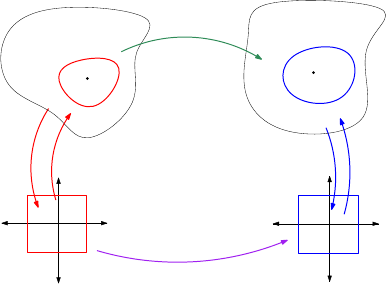Deﬁnition 13 (Smooth map between manifolds). A map F : M N between
manifolds is smooth at p M if there exists a chart (U, ϕ) around p on M and
a chart (V, ψ) around F (p) on N such that F (U) V and:
ψ F ϕ
1
: ϕ(U) (ψ F )(U),
is smooth in the usual sense.
ψ
ψ
1
ψ F ϕ
1
ϕ
ϕ
1
M
N
F
p
f(p)
This is the situation, and we require the purple map to be smooth in order for
F to be smooth. This purple map is called the transition map. In a similar
vein, if F is bijective, we say it is a diﬀeomorphism if both it and its inverse
are smooth (i.e. the transition map is a diﬀeomorphism). Diﬀeomorphism is an
equivalence relation on smooth structures.
A A bit about continuity
When we think about continuity intuitively, we often use the deﬁnition “if you
can draw the graph of the function without lifting up your pen, then it is
continuous. This of course has its limitations, though it still follows from the
formal deﬁnition:
Deﬁnition 14 (ε δ continuity). A function f : (X, d) (Y, d
0
) between
metric spaces is a continuous at a point a X if and only if for all ε > 0 there
exists a δ > 0 such that for all x X we have
d(a, x) < δ = d
0
(f(a), f(x)) < ε.
In the case f is continuous at all x X, we call f itself continuous.
Intuitively, this deﬁnition says that for any small perturbation in Y (the ε), we
can ﬁnd a small perturbation in X (the δ) which f takes inside the perturbation
in Y . That is, the perturbation in Y is controlled by that X.
10
Perhaps the word “perturbation” is not very accurate or well-thought out, but
let us make another deﬁnition to ease our notation:
Deﬁnition 15 (Open ball). Let (X, d) be a metric space and let a X. An
open ball around a of radius δ > 0 is the set
B(a; δ) := {x X : d(a, x) < δ}
Now, this deﬁnition should not be anything special, but we notice that the
d(a, x) < δ occurs in our deﬁnition for continuity, so lets simply re-write the
deﬁnition now with this new notation.
Deﬁnition 16 (Re-writing of continuity). A function f : (X, d) (Y, d
0
) be-
tween metric spaces is continuous at a point a X if and only if for all ε > 0
there exists a δ > 0 such that for all x X we have
x B(a; δ) = f(x) B(f(a); ε),
or equivalently,
f[B(a; δ)] B(f (a); ε)
Now this deﬁnition conveys a ﬁne subtlety that was not apparent when we were
staring at the distance functions. Suppose f is continuous at a X. Then
according to the above newly-notated deﬁnition we have that for any ε > 0 we
have a δ > 0 such that
B(a; δ) f
1
[B(f(a); ε)] := {x X : f (x) B(f (a); ε)},
that is, the pre-image of any ε-ball around f (a) is a neighbourhood of a (by
“neighbourhood” here, we mean a subset containing a and an open ball around
a of some radius).
Suppose N
0
is a neighbourhood of f(a) that is, it contains an ε-ball around
f(a). Then we see that by virtue of the observation above, the pre-image of N
0
is a neighbourhood N of a. In conclusion, we have:
f continuous at a = pre-images of neighbourhoods of f (a) are neighbourhoods of a.
Now we ask outselves, “what about the converse?” Well, let’s suppose that
pre-images of neighbourhoods of f(a) are neighbourhoods of a:
Let ε > 0 and consider the ball N
0
:= B(f(a); ε) around f(a). It is true that
every open ball is a neighbourhood of each of its points (exercise!), so by our
assumption, the pre-image N := f
1
[B(f(a); ε)] is a neighbourhood of a. By
deﬁnition of a neighbourhood, N contains a ball B(a; δ) around a, and it is clear
then that f(N ) N
0
and hence f is continuous at a. Summarily:
11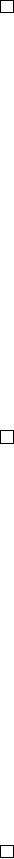Theorem 2 (Neighbourhood deﬁnition). A function f : (X, d) (Y, d
0
) is
continuous at a X if and only if pre-images of neighbourhoods of f(a) are
neighbourhoods of a.
Proof. The proof is embedded in the text above.
Now we are not done here, though we might as well be. Consider the following
generalization of an open ball:
Deﬁnition 17 (Open subset). A subset O X of a metric space (X, d) is an
open set if and only if it is a neighbourhood of each of its points.
For example, an open ball is an open set, or even the arbitrary union of open
sets. Indeed:
Theorem 3. A subset O X of a metric space (X, d) is open if and only if it
is a union of open balls.
Proof. Suppose O is open. Then for each x O there exists an open ball
B(x; δ
x
) O. It follows that O =
S
xO
B(x; δ
x
). On the other hand, suppose
O is a union of open balls. Each x O is in an open ball, just call it B, which
is also an open set (by an exercise). Hence we have a B(x; δ) B. Hence O is
a neighbourhood of each x O, and is open.
With this in mind, we want to phrase continuity in terms of open sets, rather
than open balls or neighbourhoods. So we ﬁnally consider the following charac-
terization:
Theorem 4 (Open set deﬁnition). A function f : (X, d) (Y, d
0
) is continuous
if and only if the pre-images of open sets in Y are open in X.
Proof. Suppose f is continuous and let O Y be an open set in Y . For each
x f
1
[O] we have that O is a neighbourhood of f(x). By continuity of f and
Theorem 2, f
1
[O] is a neighbourhood of x. Hence f
1
[O] is open in X.
On the other hand, suppose the pre-image of each open set in Y is open in X.
Let a X, and our goal will now be to show that f is continuous at a. Let N
0
be
a neighbourhood of f (a), then it contains an open ball B with f (a) B N
0
.
Because B is open, its pre-image N := f
1
[B] is also open by assumption, and
thus is a neighbourhood of a. Hence f
1
[N
0
] contains a neighbourhood of a
Thus f is continuous at a. Because a was arbitrary, f is continuous.
Now the conclusion here is that continuity only depends on the metric as much
as it depends on the open sets. The metric gives rise to open balls which give
rise to neighbourhoods which give rise to open sets, all of which we can deﬁne
continuity by.
12
In the ﬁeld of topology, we ignore the metric and open balls, but deﬁne the
system of neighbourhoods or open sets of a space. We use observations about
open sets in metric spaces to characterize open sets (the collection of which we
call a topology), and it just so happens our characterization of open sets allow
for more general spaces than metric spaces. That is to say, every metric space
is a topological space, but not the other way around. In a topological space,
Theorem 4 functions as the deﬁnition of continuity.
13